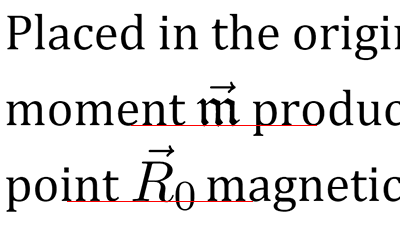# LaTeX equations for web

## Online editorImage URL:

## Examples

### Integrals, roots and borders

$$\boxed{ \int\limits_{-\infty}^{\infty} e^{-x^2} \, dx = \sqrt{\pi} }$$
\boxed{ \int\limits_{-\infty}^{\infty} e^{-x^2} \, dx = \sqrt{\pi} }

### Limits and sums

$$\gamma \overset{\text{def}}{=} \lim\limits_{n \to \infty} \left( \sum\limits_{k=1}^n {1 \over k} - \ln n \right) \approx 0.577$$
\gamma \overset{\text{def}}{=} \lim\limits_{n \to \infty} \left( \sum\limits_{k=1}^n {1 \over k} - \ln n \right) \approx 0.577

### Multiline equations

\begin{align*} y &= x^4 + 4 =\\ &= (x^2+2)^2 - 4x^2 \le\\ &\le (x^2+2)^2 \end{align*}
\begin{align*} y &= x^4 + 4 =\\ &= (x^2+2)^2 - 4x^2 \le\\ &\le (x^2+2)^2 \end{align*}

### Matrices

$$A_{m,n} = \begin{pmatrix} a_{1,1} & a_{1,2} & \cdots & a_{1,n} \\ a_{2,1} & a_{2,2} & \cdots & a_{2,n} \\ \vdots & \vdots & \ddots & \vdots \\ a_{m,1} & a_{m,2} & \cdots & a_{m,n} \end{pmatrix}$$
A_{m,n} = \begin{pmatrix} a_{1,1} & a_{1,2} & \cdots & a_{1,n} \\ a_{2,1} & a_{2,2} & \cdots & a_{2,n} \\ \vdots & \vdots & \ddots & \vdots \\ a_{m,1} & a_{m,2} & \cdots & a_{m,n} \end{pmatrix}

### Continued fractions

$$e = 2 + \cfrac{1}{ 1 + \cfrac{1}{ 2 + \cfrac{2}{ 3 + \cfrac{3}{ 4 + \cfrac{4}{\ldots} }}}}$$
e = 2 + \cfrac{1}{ 1 + \cfrac{1}{ 2 + \cfrac{2}{ 3 + \cfrac{3}{ 4 + \cfrac{4}{\ldots} }}}}

### Picture environment

$$\begin{picture}(76,20) \put(0,0){A} \put(69,0){B} \put(14,3){\line(1,0){50}} \put(39,3){\vector(0,1){15}} \put(14,3){\circle*{2}} \put(64,3){\circle*{2}} \end{picture}$$
\begin{picture}(76,20) \put(0,0){$A$} \put(69,0){$B$} \put(14,3){\line(1,0){50}} \put(39,3){\vector(0,1){15}} \put(14,3){\circle*{2}} \put(64,3){\circle*{2}} \end{picture}

### Diagrams xy-pic

$$\xymatrix{ A \ar[r]^f \ar[d]_g & B \ar[d]^{g'} \\ D \ar[r]_{f'} & C }$$
\xymatrix{ A \ar[r]^f \ar[d]_g & B \ar[d]^{g'} \\ D \ar[r]_{f'} & C }

### TikZ graphics

$$\begin{tikzpicture}\small \def\r{1.8} \coordinate[label=A] (A) at (0.5*\r,0.8*\r); \coordinate[label=below:B] (B) at (-\r,0); \coordinate[label=below:C] (C) at (\r,0); \draw[thin] (A) -- node[above] {c} node[pos=0.03,below,inner sep=4] {\alpha} (B) -- (C) -- node[right] {b} (A); \end{tikzpicture}$$
\begin{tikzpicture}\small \def\r{1.8} \coordinate[label=$A$] (A) at (0.5*\r,0.8*\r); \coordinate[label=below:$B$] (B) at (-\r,0); \coordinate[label=below:$C$] (C) at (\r,0); \draw[thin] (A) -- node[above] {$c$} node[pos=0.03,below,inner sep=4] {$\alpha$} (B) -- (C) -- node[right] {$b$} (A); \end{tikzpicture}

### TikZ plots

$$\begin{tikzpicture}[domain=0:2] \draw[very thin] (-0.1,-0.1) grid (2.1,2.1); \draw[->] (-0.2,0)--(2.2,0) node[right] {x}; \draw[->] (0,-0.2)--(0,2.2) node[above] {y}; \draw plot (\x,{sin(\x r)}) node[right] {y=\sin x}; \draw[color=blue] plot (\x,\x) node[right] {y=x}; \end{tikzpicture}$$
\begin{tikzpicture}[domain=0:2] \draw[very thin] (-0.1,-0.1) grid (2.1,2.1); \draw[->] (-0.2,0)--(2.2,0) node[right] {$x$}; \draw[->] (0,-0.2)--(0,2.2) node[above] {$y$}; \draw plot (\x,{sin(\x r)}) node[right] {$y=\sin x$}; \draw[color=blue] plot (\x,\x) node[right] {$y=x$}; \end{tikzpicture}

## FAQ

### What is “LaTeX”?

LaTeX is a computer typesetting system for creating complex documents. It is widely used in scientific publications, especially in physics and math. For more details, refer to Wikipedia.

### What does this website do exactly?

This website converts mathematical expressions into web-ready pictures. You don’t need to create them in a graphical editor and to upload somewhere. You can easily add pictures to discussions on blogs or forums, or share links via messengers.

### Is it free to use?

Yes, it is free to use, as long as there is a reasonable load on the service. If your requests start to obstruct other users, your access may be blocked.

### Is there a guarantee that the service won’t stop working?

No, there is no guarantee. However, I personally use it on my own websites and have no plans to shut it down.

### How are the formulas converted into images?

The server has TeX Live installed, which works in conjunction with modern web technologies.

### Cyrillic and hieroglyphic characters are not displayed in the formulas!

Perhaps the \text command can help you. For example, Q_\text{плавления}>0 is displayed correctly: $$Q_\text{плавления}>0$$.

### How to include LaTeX packages? I want images with chemical formulas and musical notations!

When creating images, a minimal set of packages is included. If you need a specific package, please write me an email. Don’t forget to explain how the package will be useful to other users.

### Do I have to type all formulas in this editor?

Not necessarily. If you have only few formulas, it’s convenient to type them directly in the equation editor. For longer texts, you can use the Upmath editor with LaTeX and Markdown support. Additionally, I have developed a script for math-rich websites that enables you to use LaTeX directly in the HTML source code.

## Embedding into websites

Authors of mathematical text can write LaTeX expressions directly in HTML code. To have them automatically converted, enclose the expressions within dollar signs $$...$$, and include the following script:

<script src="//i.upmath.me/latex.js"></script>

Here is a sample of HTML code:

<p>Placed in the origin, magnetic moment $$\vec{\mathfrak{m}}$$ produces at point $$\vec{R}_0$$ magnetic vector potential</p> <p>$$\vec{A} = {\vec{\mathfrak{m}} \times \vec{R}_0 \over R_0^3}.$$(1)</p>

The rendered result:

Placed in the origin, magnetic moment $$\vec{\mathfrak{m}}$$ produces at point $$\vec{R}_0$$ magnetic vector potential

$$\vec{A} = {\vec{\mathfrak{m}} \times \vec{R}_0 \over R_0^3}.$$(1)

In modern browsers, the script loads pictures in vector format SVG and aligns the formula’s baseline with the surrounding text:This service powers my blog on theoretical physics.